# Karnataka SSLC Board (KSEEB) Question Paper for Class 10th Maths 2017 In PDF

## Karnataka SSLC or Class 10 Maths Question Paper 2017 with Solutions – Free Download

KSEEB question papers 2017 Class 10 Maths are available at BYJU’S with answers to help the students in verifying their solutions after solving the SSLC 2017 question paper. Students can download the pdf of SSLC question paper 2017 Karnataka to practise the questions, as a part of the preparation for the examination. Also, they can access the SSLC model question papers in the pdf format, so that students can easily download. All the recent years, KSEEB SSLC Class 10 Maths question papers are given in a downloadable pdf format here. However, KSEEB Maths question papers 2017, will help the students to practice different types of questions of Class 10 Maths.

### KSEEB Class 10 Maths Question Paper Solution 2017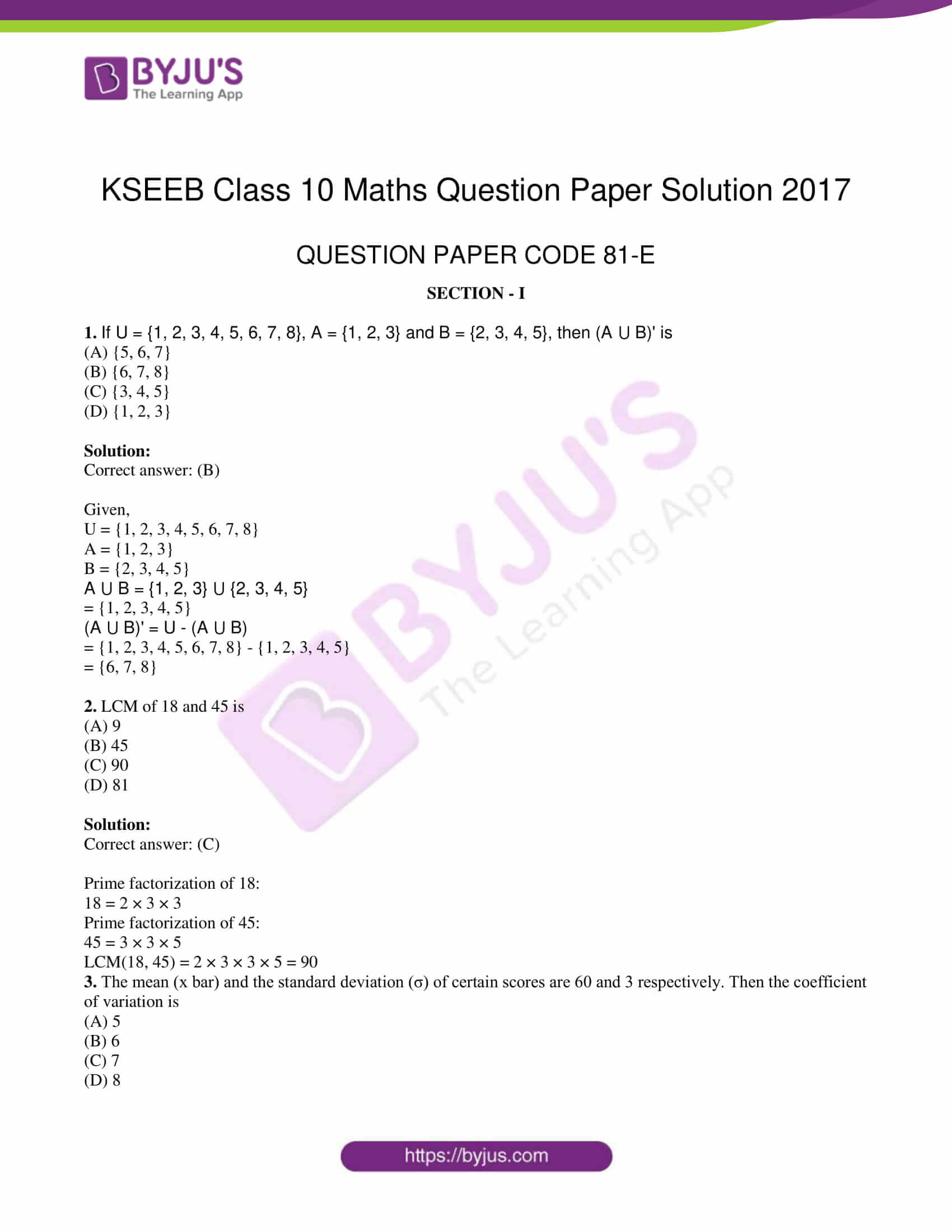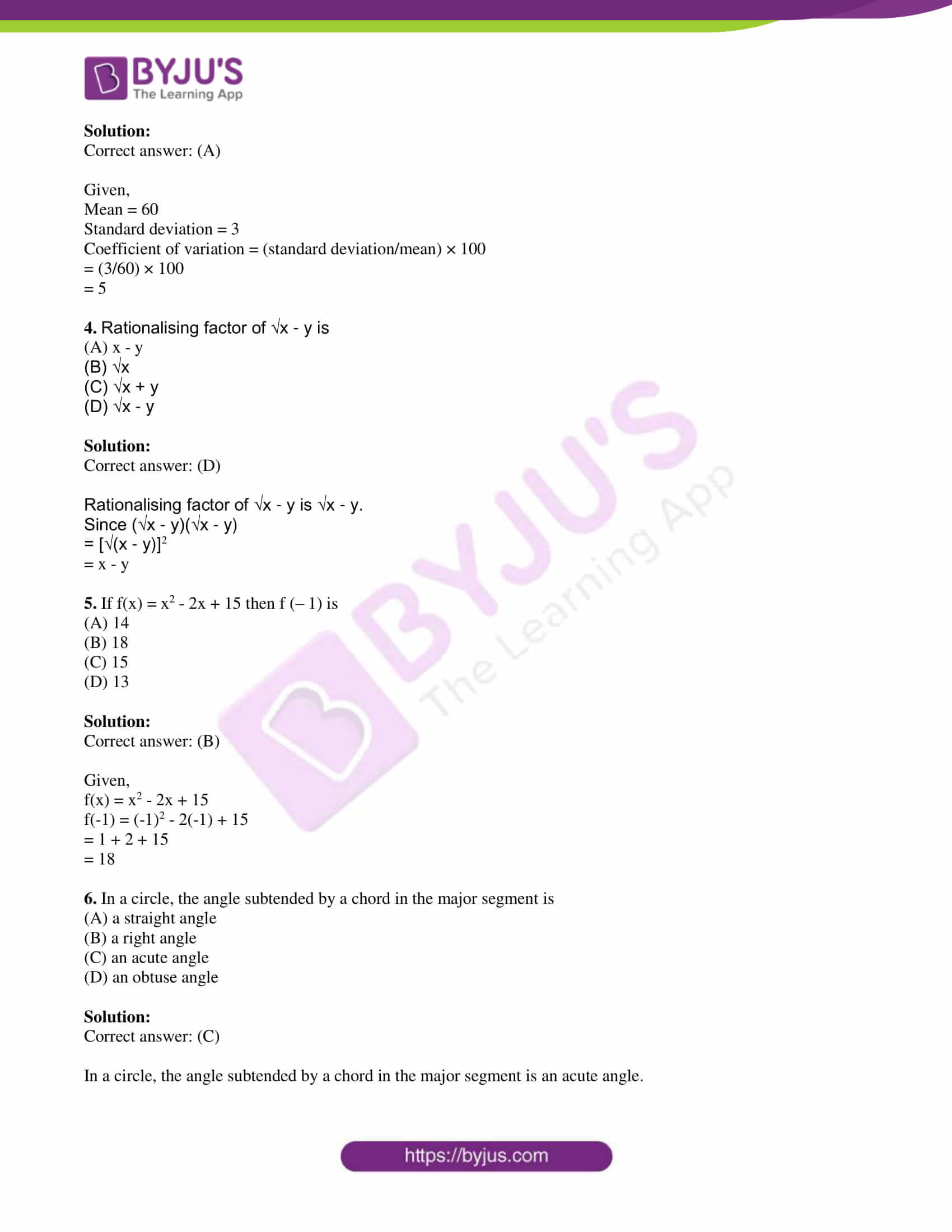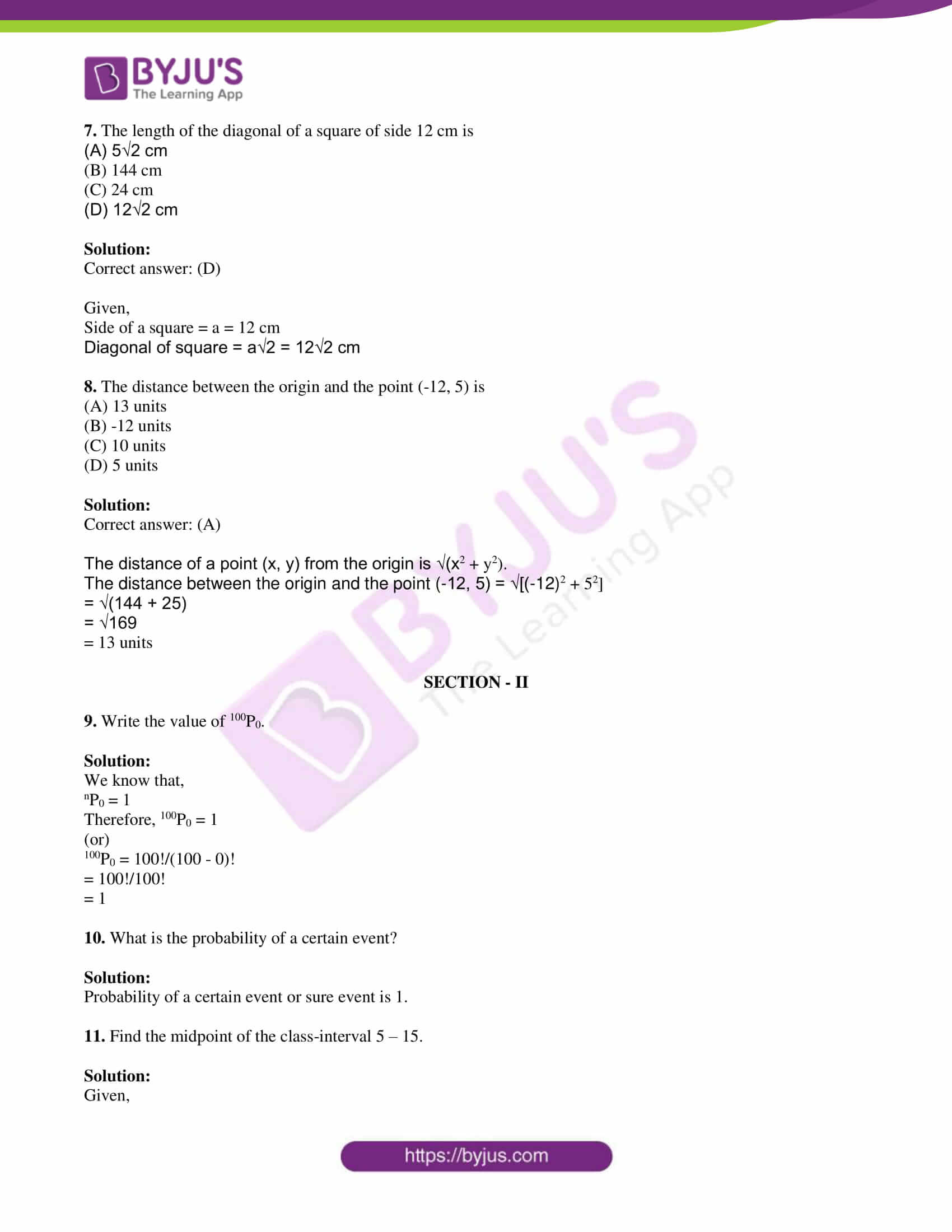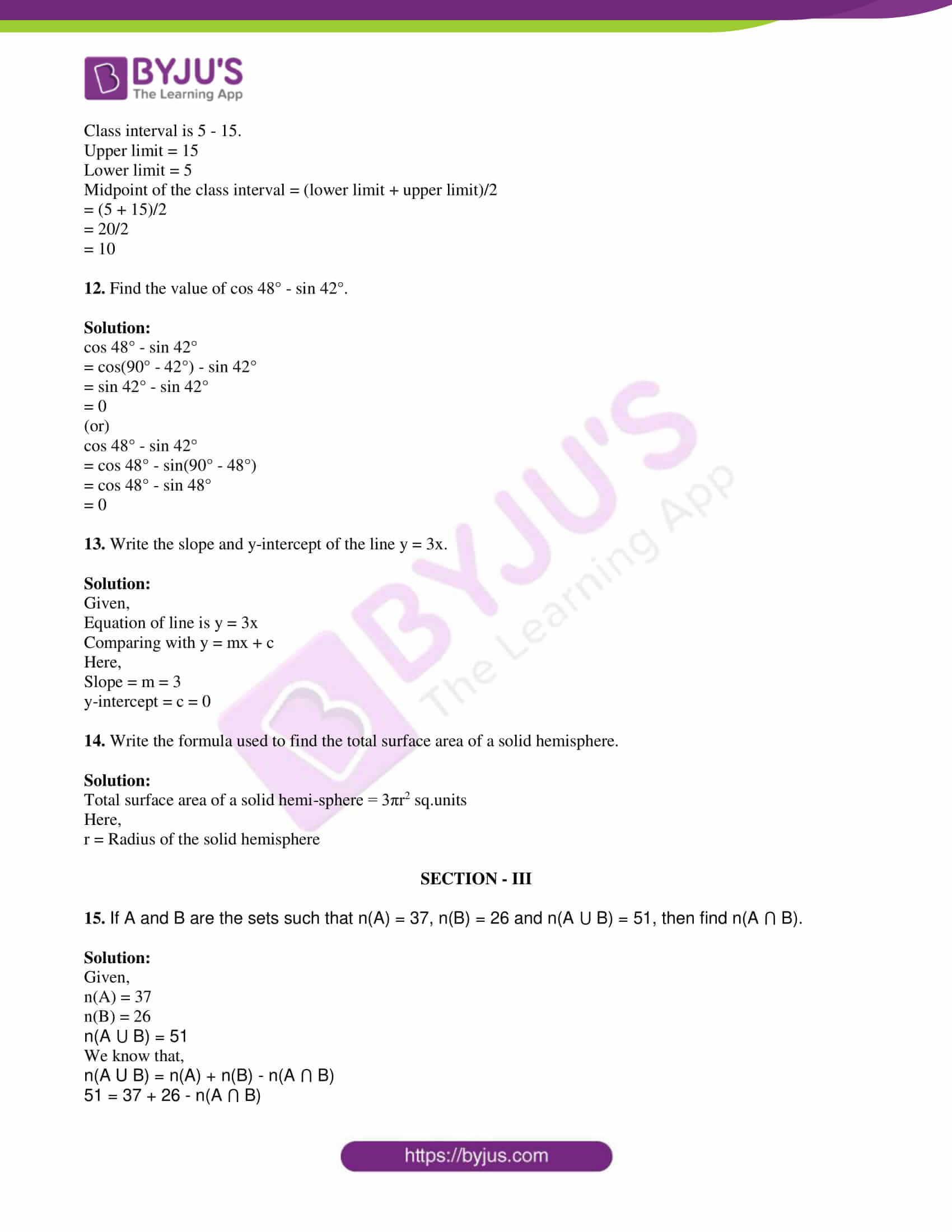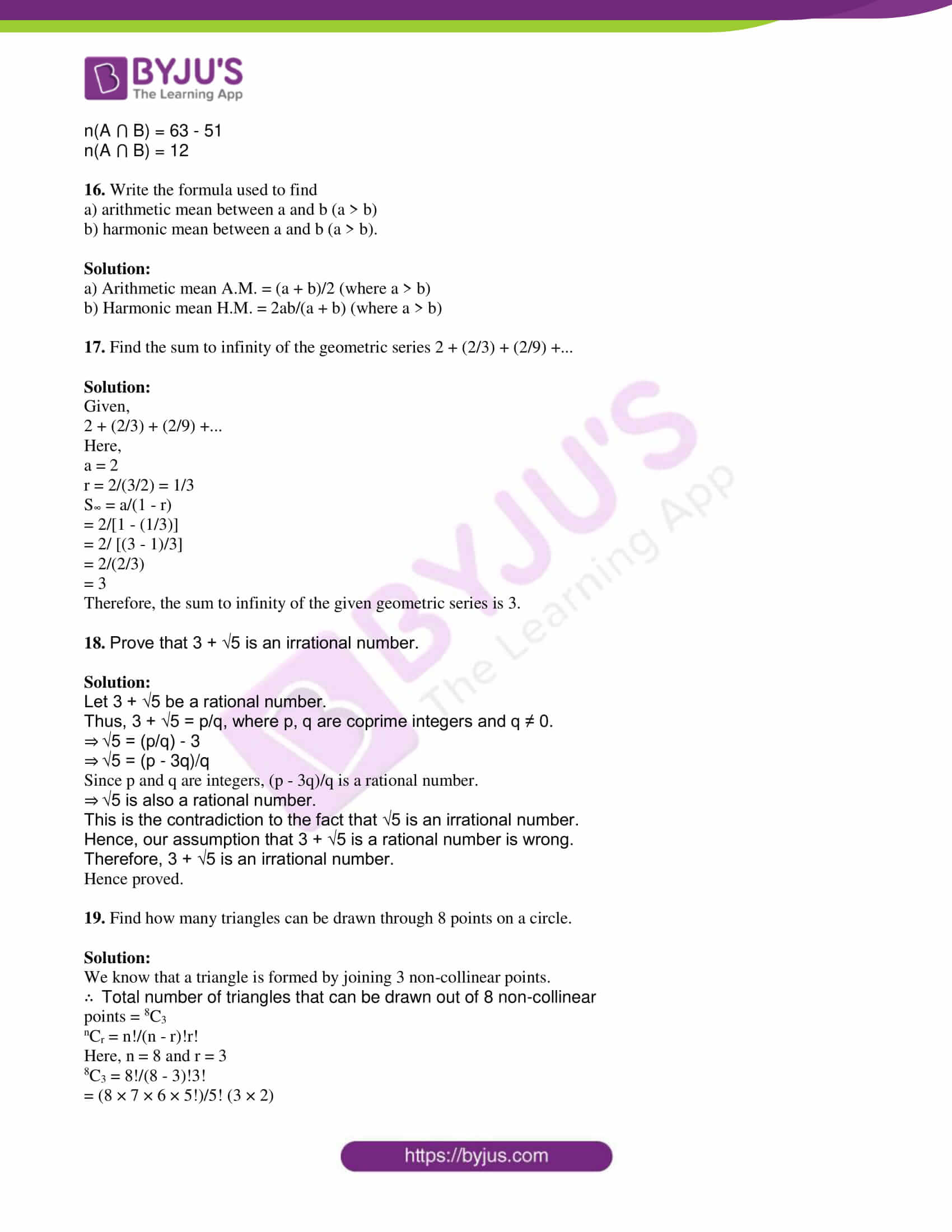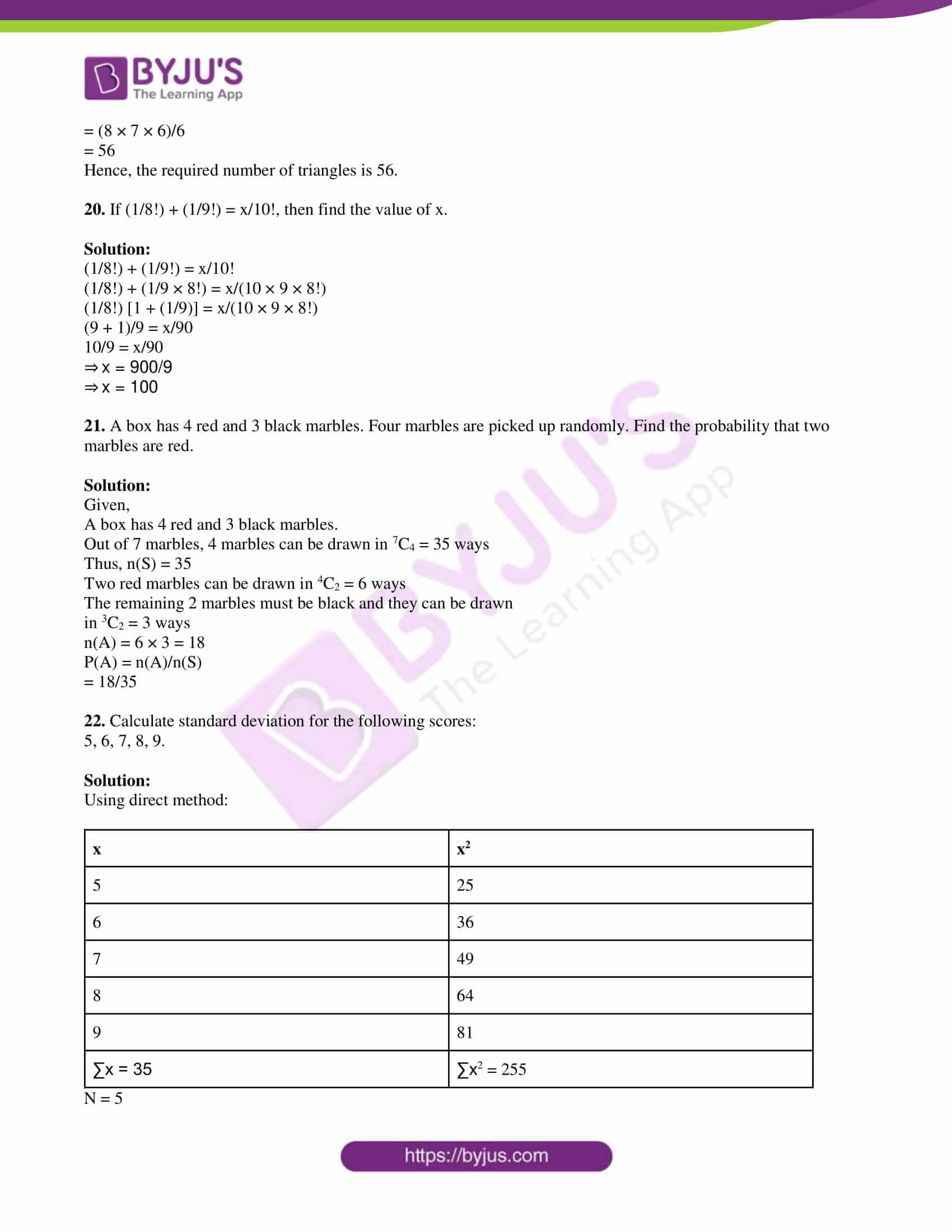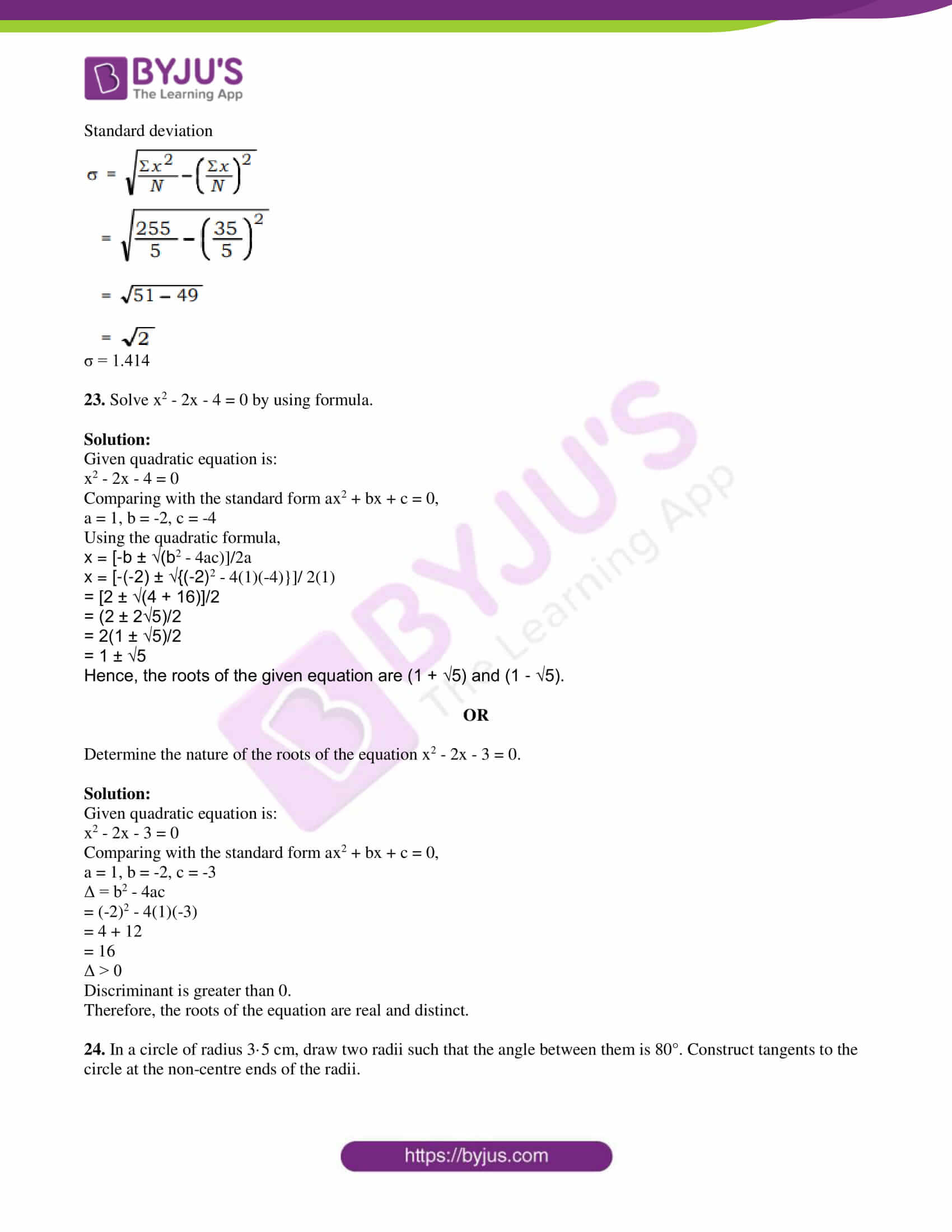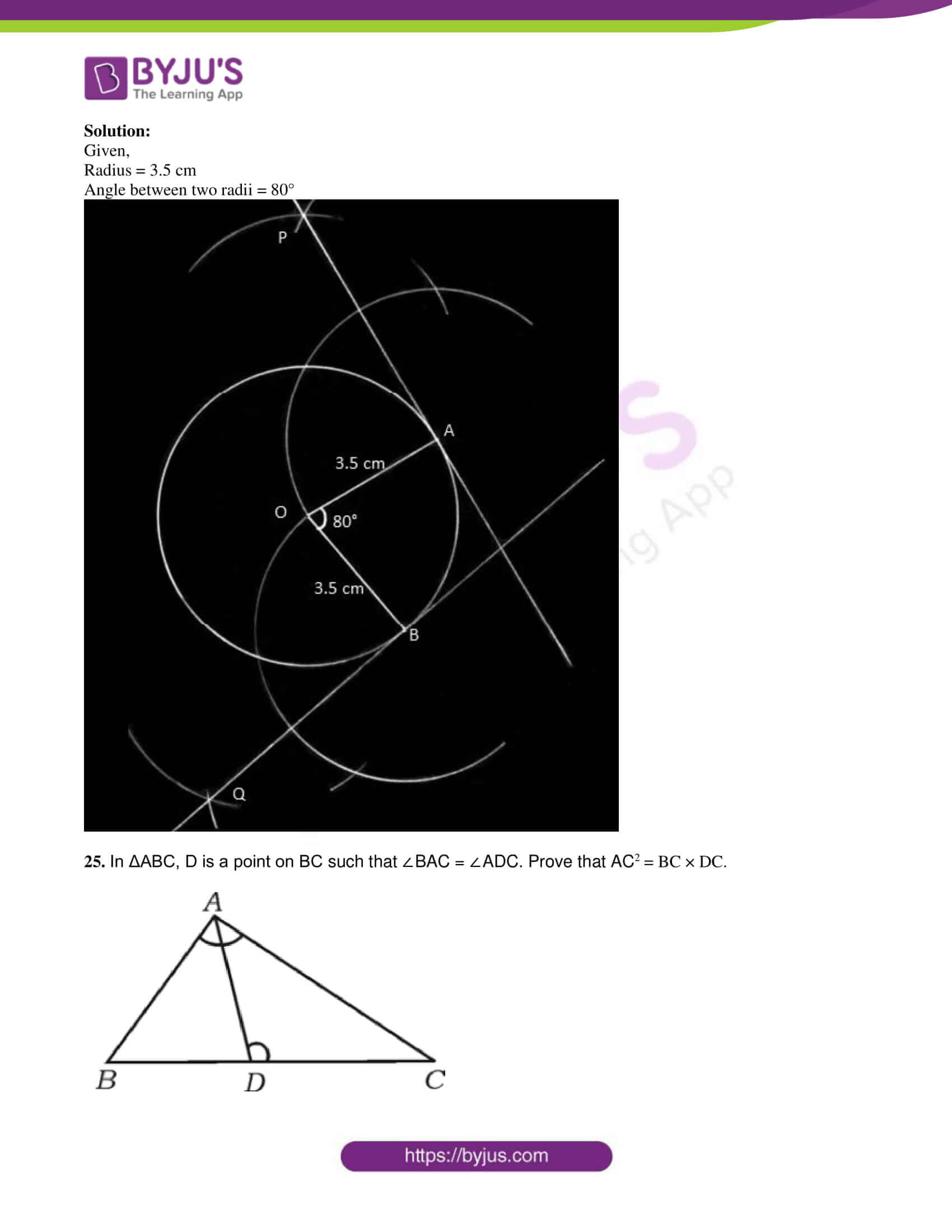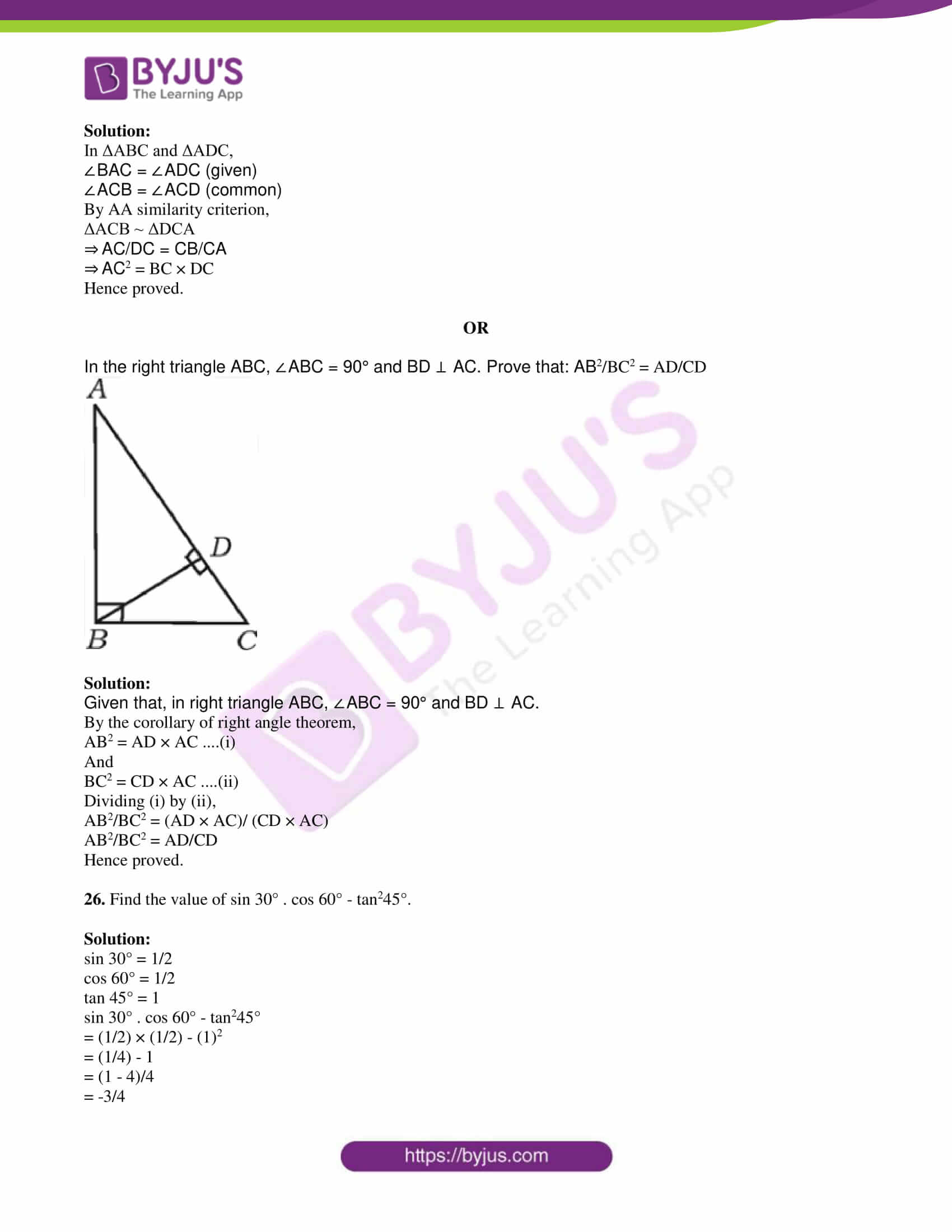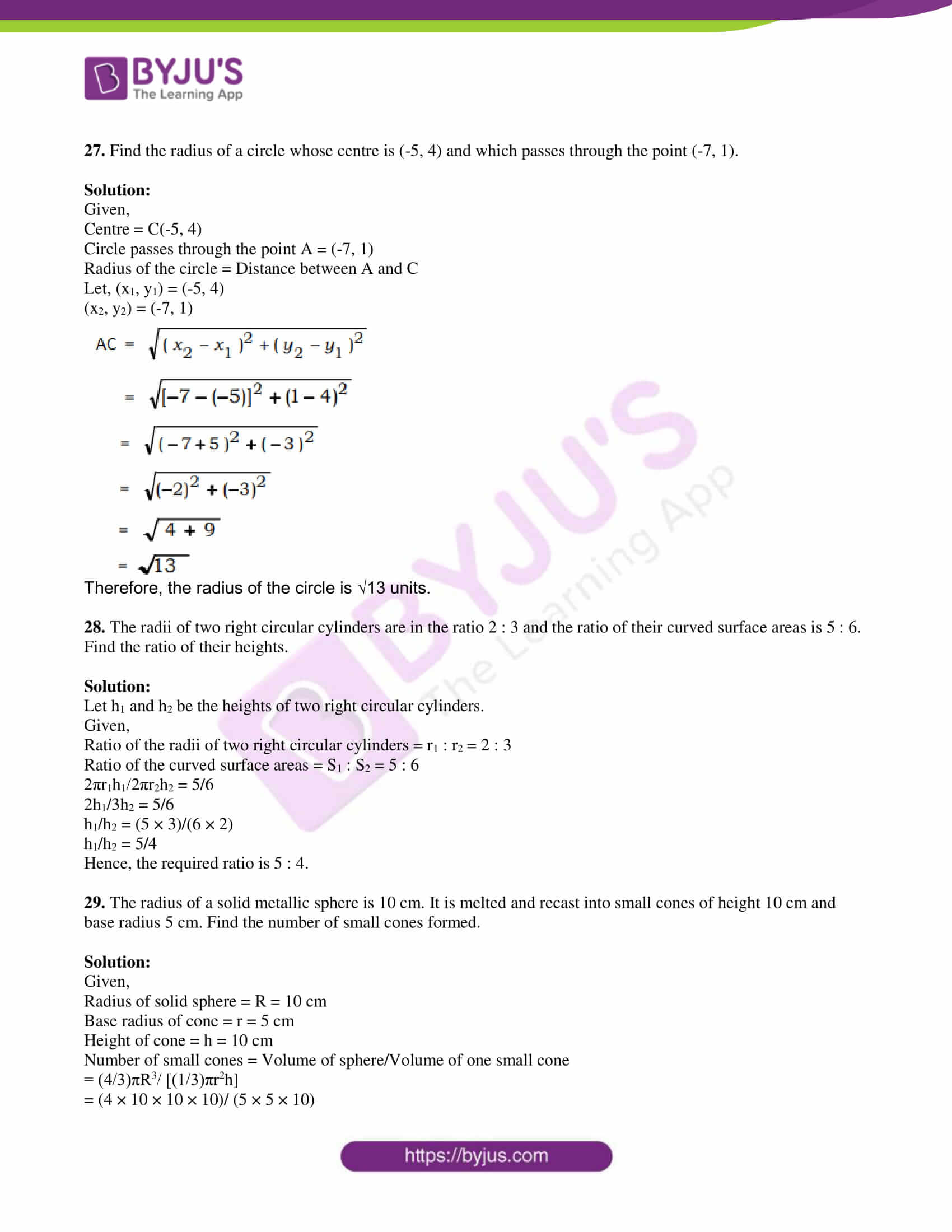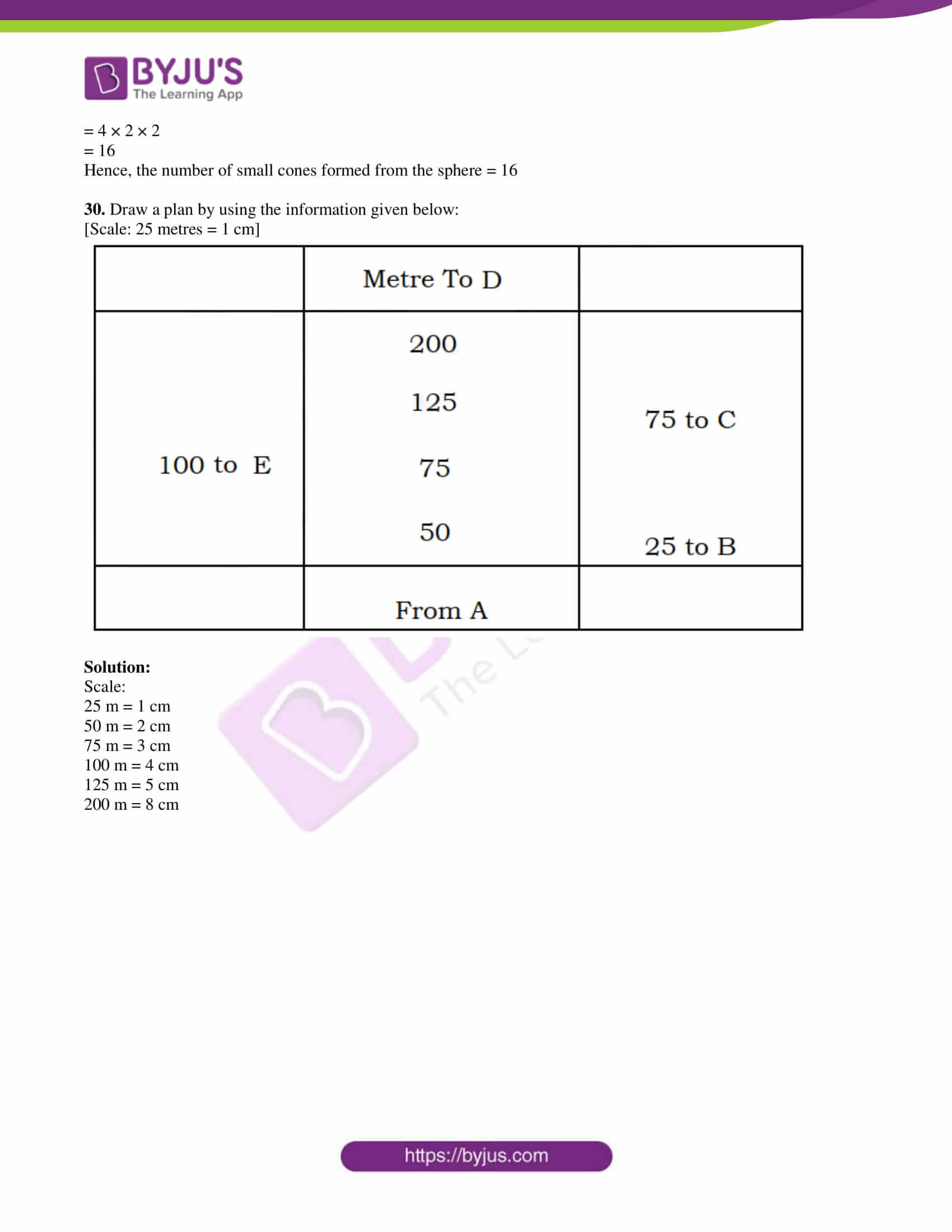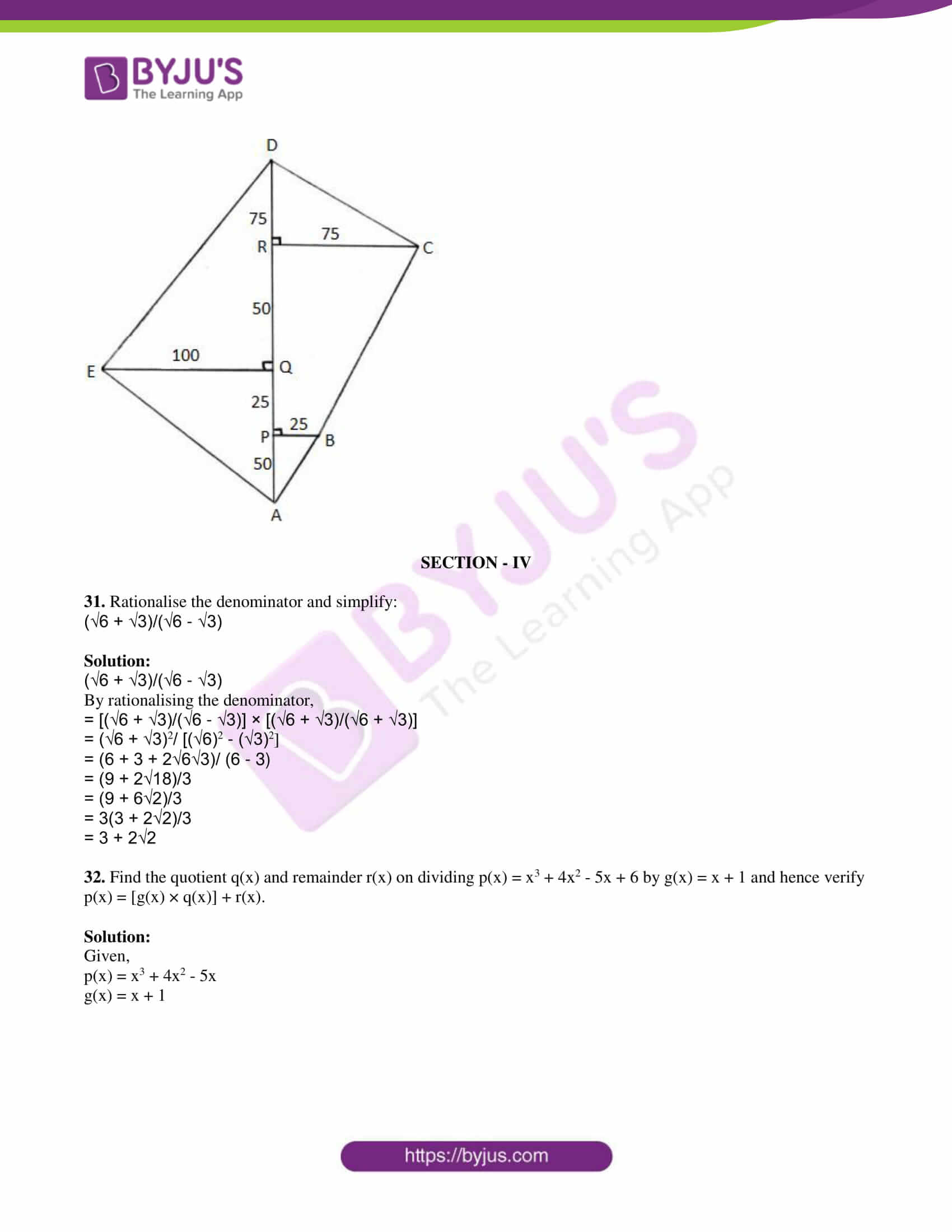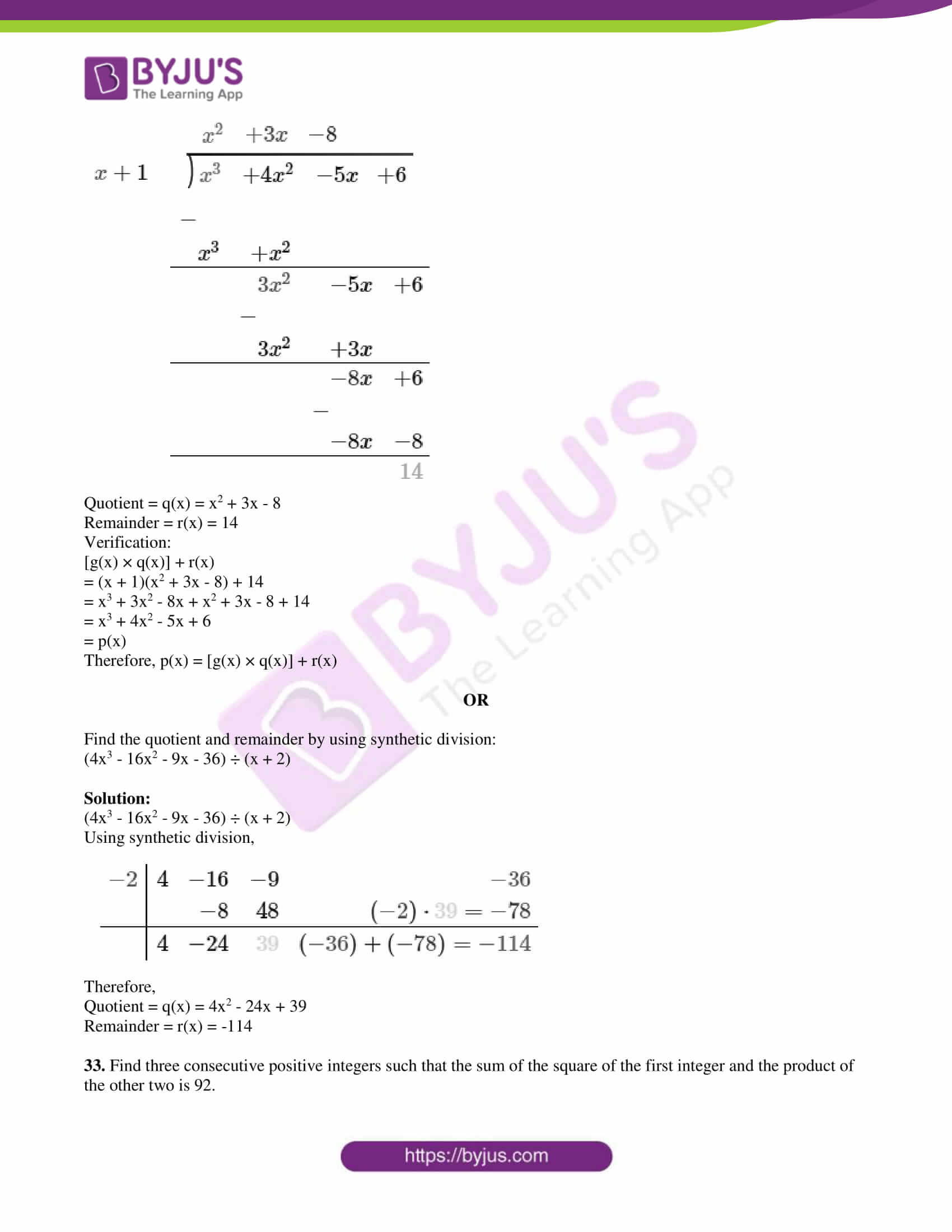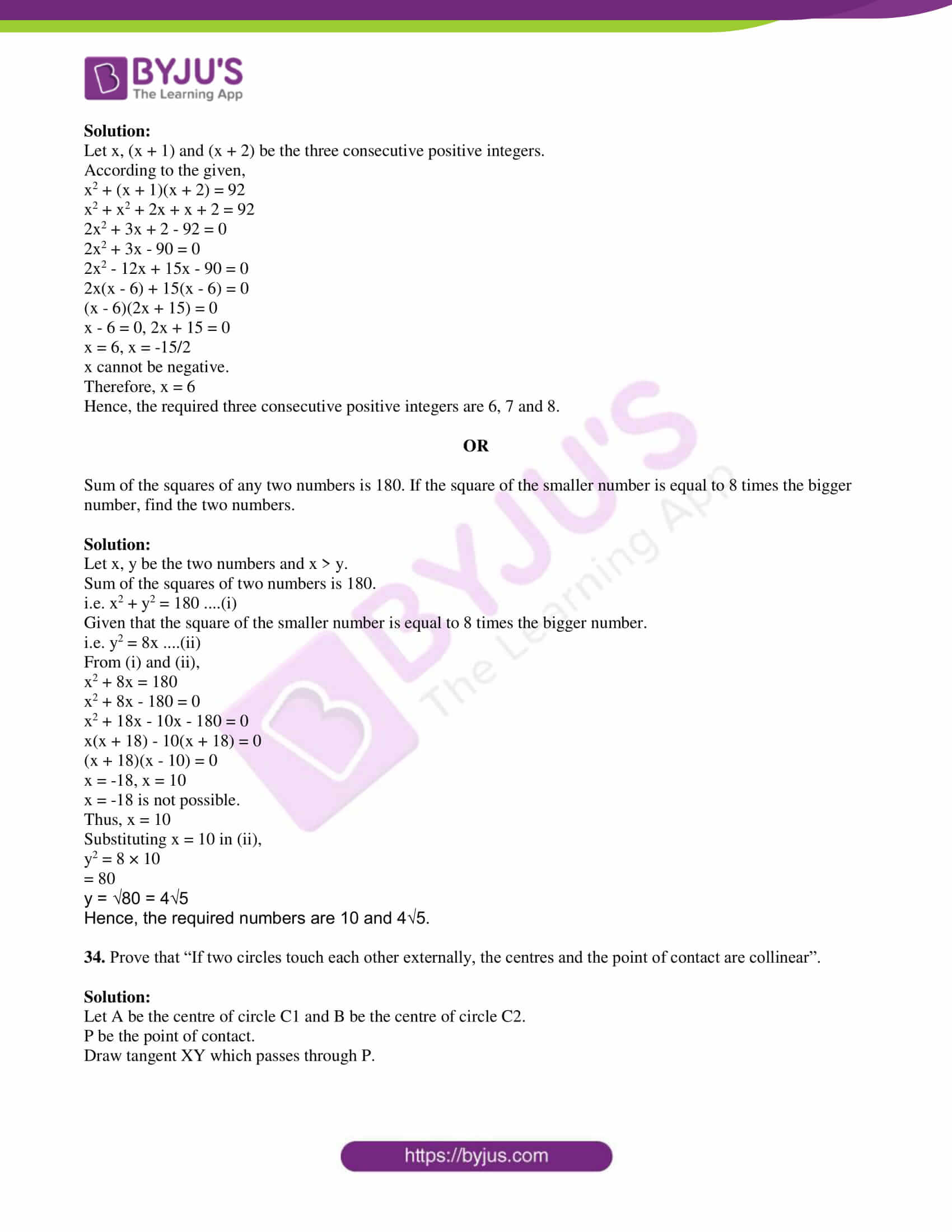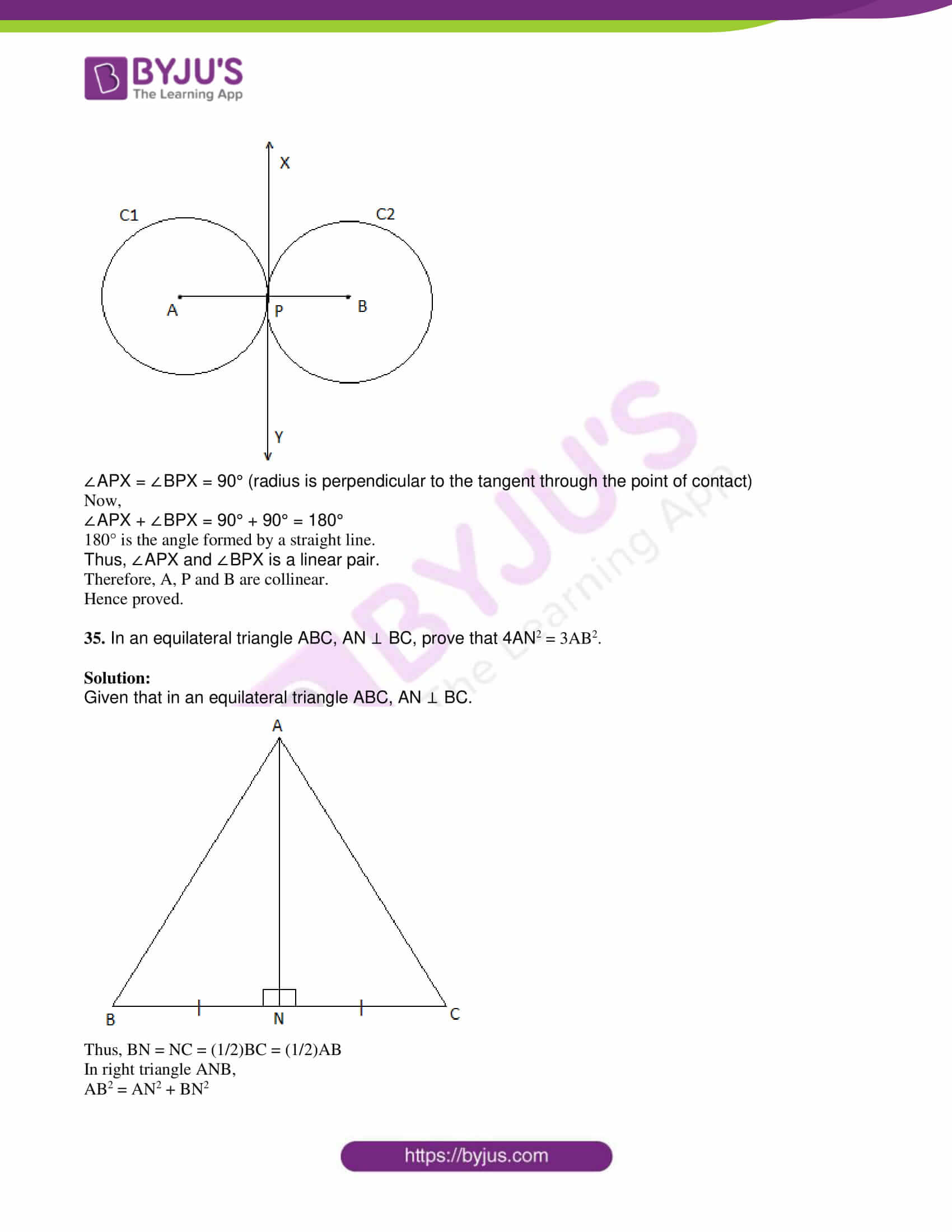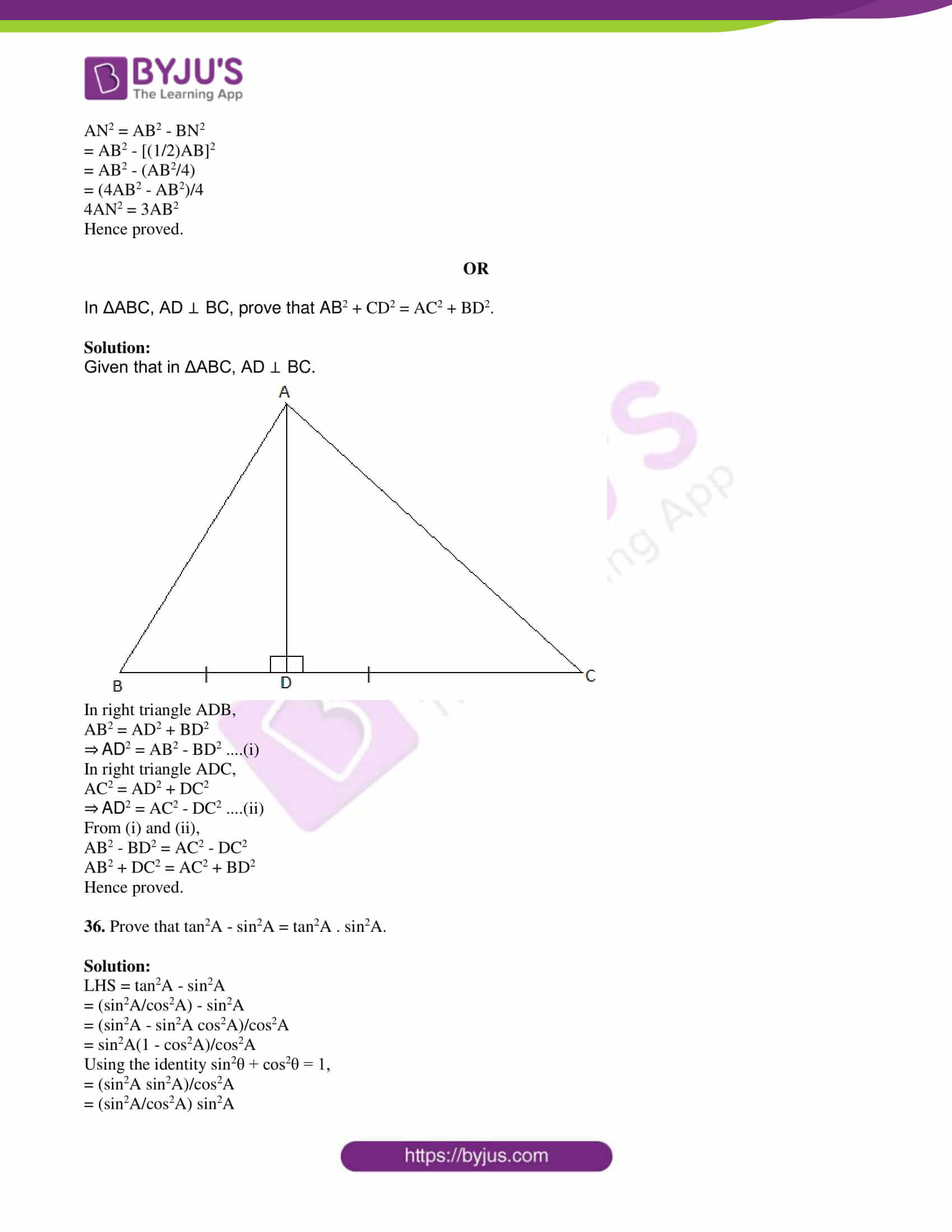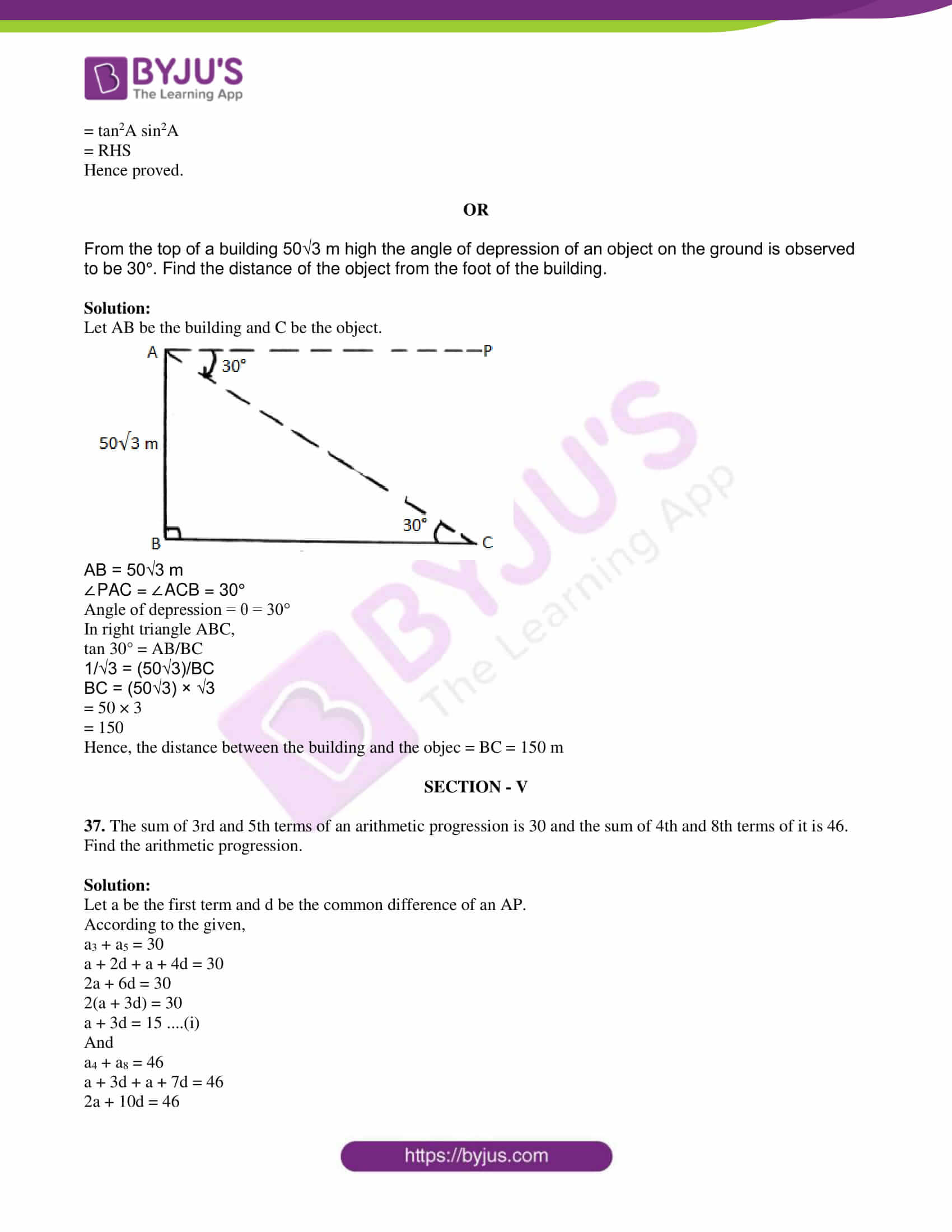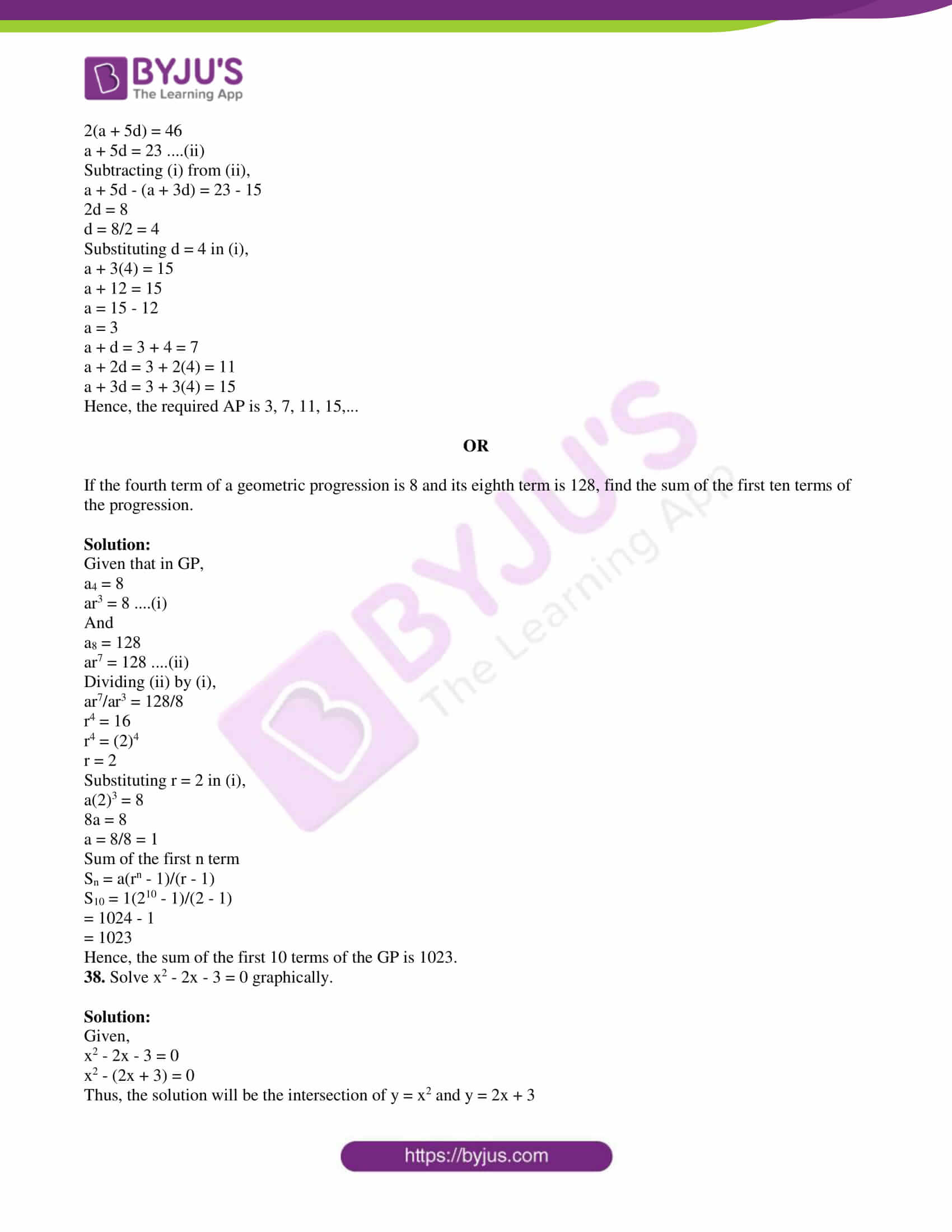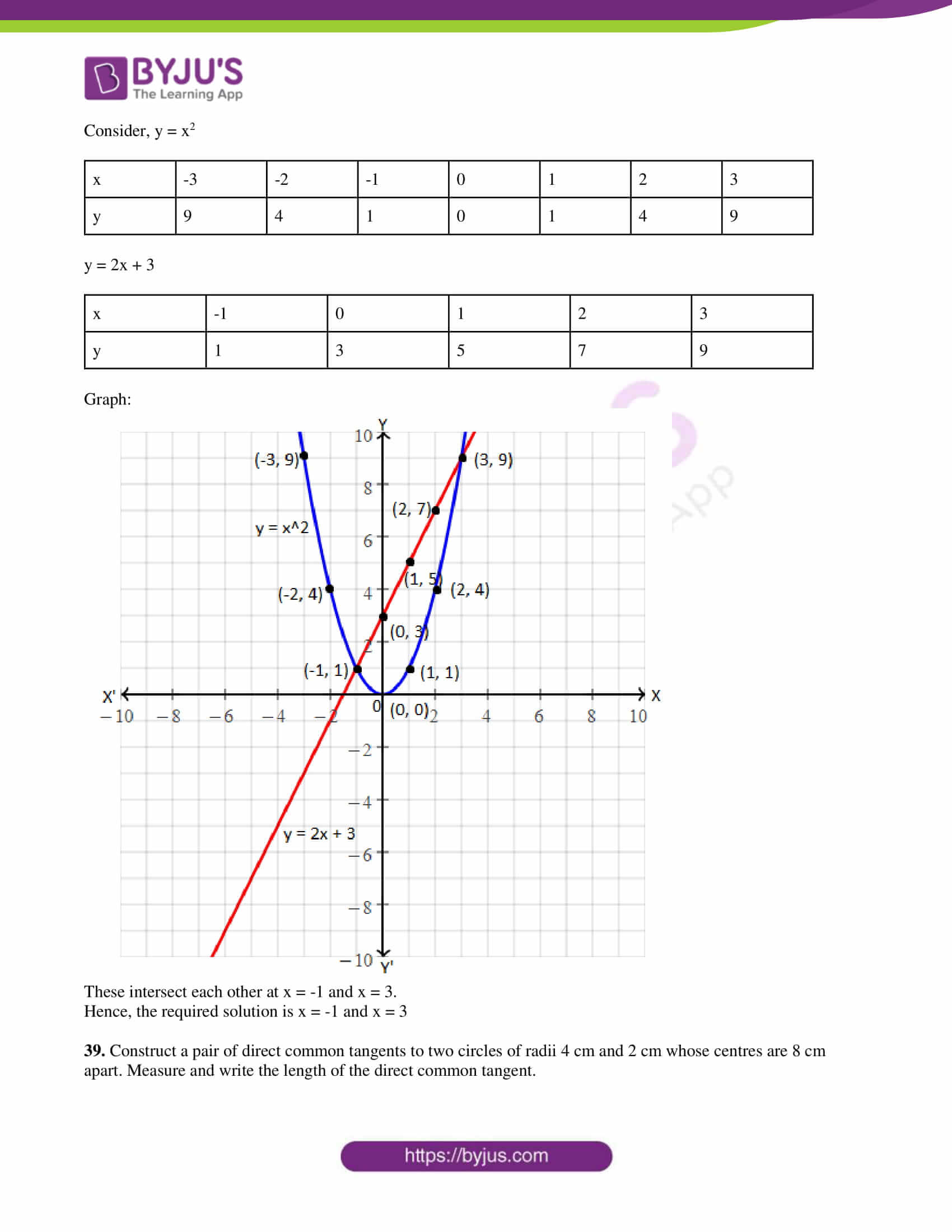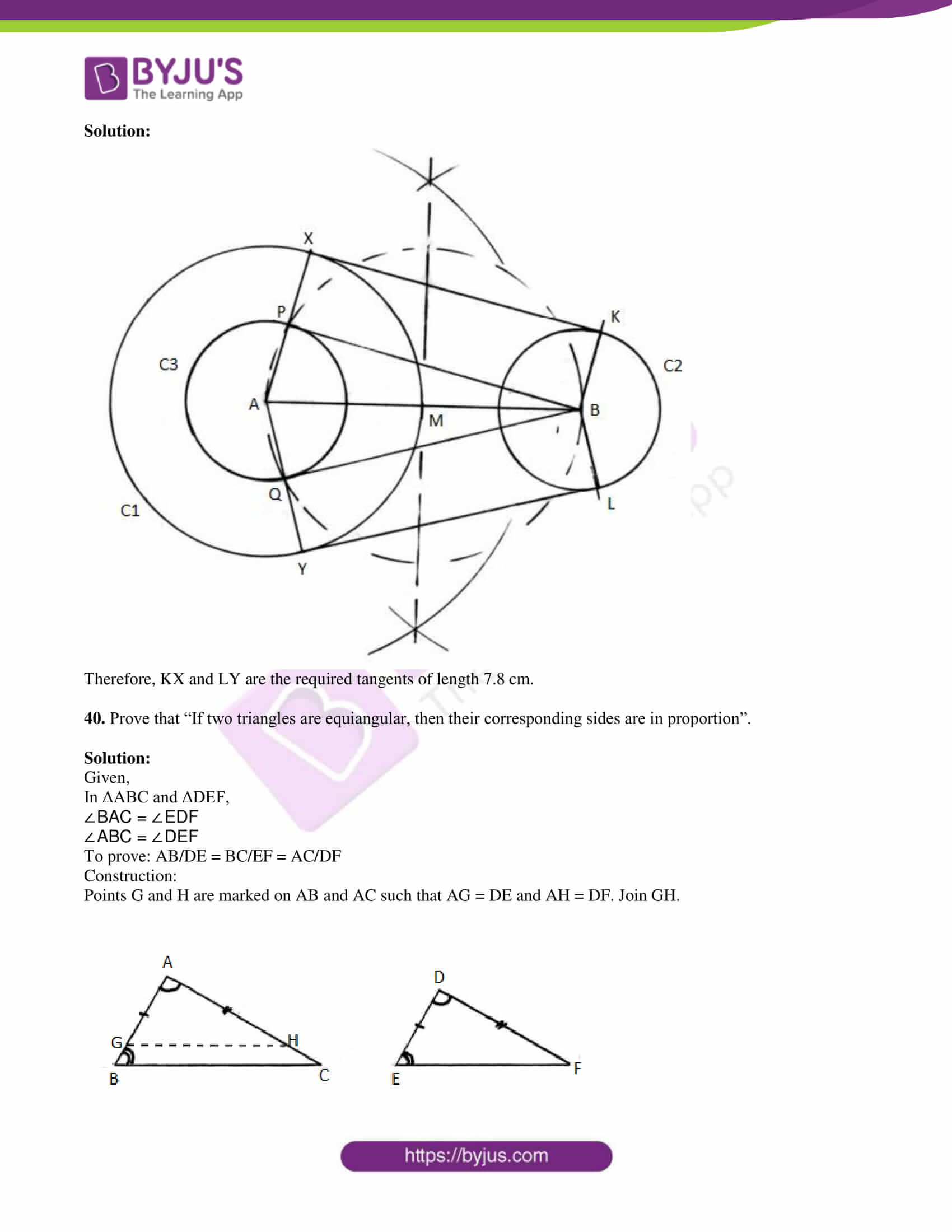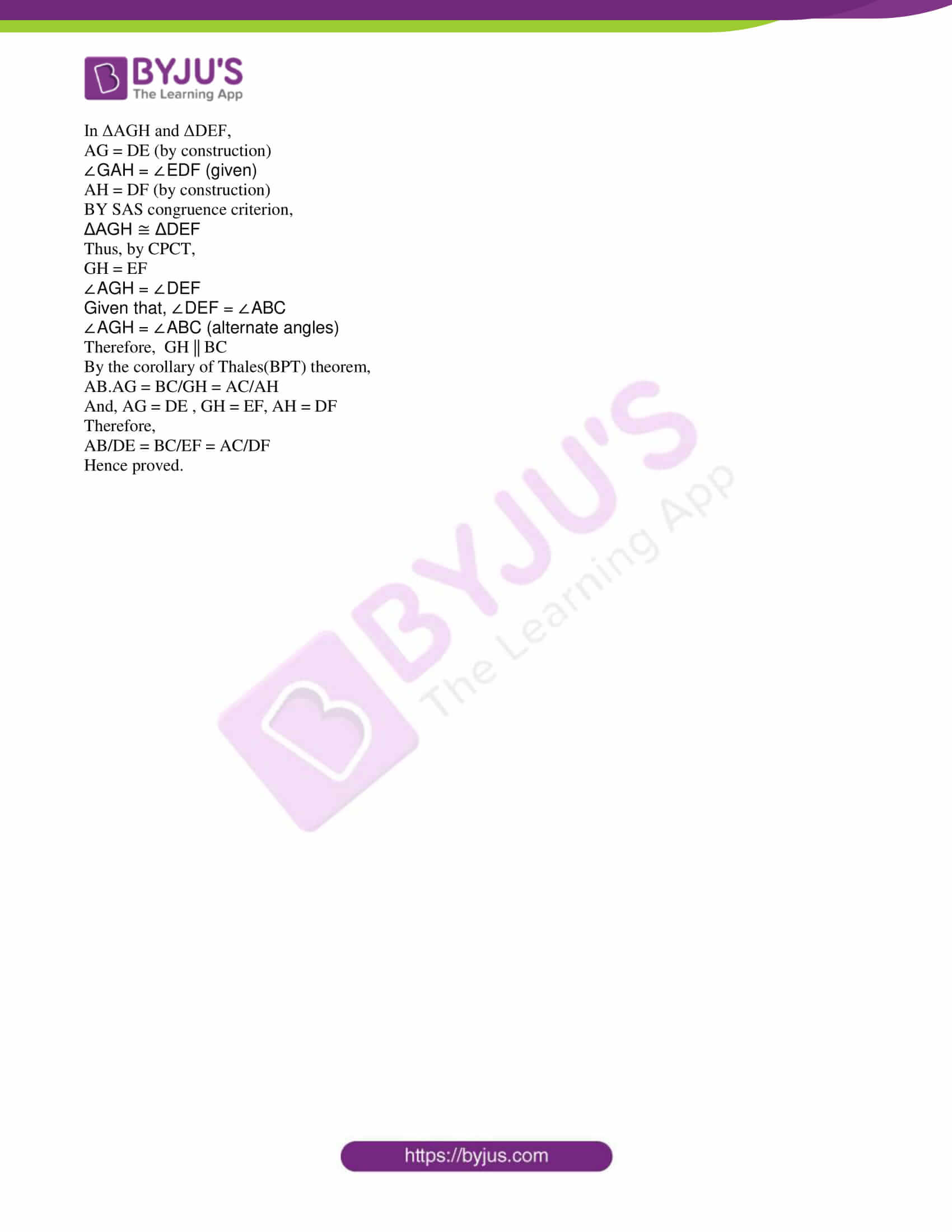### QUESTION PAPER CODE 81-E

SECTION – I

1. If U = {1, 2, 3, 4, 5, 6, 7, 8}, A = {1, 2, 3} and B = {2, 3, 4, 5}, then (A ⋃ B)’ is

(A) {5, 6, 7}

(B) {6, 7, 8}

(C) {3, 4, 5}

(D) {1, 2, 3}

Solution:

Given,

U = {1, 2, 3, 4, 5, 6, 7, 8}

A = {1, 2, 3}

B = {2, 3, 4, 5}

A ⋃ B = {1, 2, 3} ⋃ {2, 3, 4, 5}

= {1, 2, 3, 4, 5}

(A ⋃ B)’ = U – (A ⋃ B)

= {1, 2, 3, 4, 5, 6, 7, 8} – {1, 2, 3, 4, 5}

= {6, 7, 8}

2. LCM of 18 and 45 is

(A) 9

(B) 45

(C) 90

(D) 81

Solution:

Prime factorization of 18:

18 = 2 × 3 × 3

Prime factorization of 45:

45 = 3 × 3 × 5

LCM(18, 45) = 2 × 3 × 3 × 5 = 90

3. The mean (x bar) and the standard deviation (σ) of certain scores are 60 and 3 respectively. Then the coefficient of variation is

(A) 5

(B) 6

(C) 7

(D) 8

Solution:

Given,

Mean = 60

Standard deviation = 3

Coefficient of variation = (standard deviation/mean) × 100

= (3/60) × 100

= 5

4. Rationalising factor of √x – y is

(A) x – y

(B) √x

(C) √x + y

(D) √x – y

Solution:

Rationalising factor of √x – y is √x – y.

Since (√x – y)(√x – y)

= [√(x – y)]2

= x – y

5. If f(x) = x2 – 2x + 15 then f (– 1) is

(A) 14

(B) 18

(C) 15

(D) 13

Solution:

Given,

f(x) = x2 – 2x + 15

f(-1) = (-1)2 – 2(-1) + 15

= 1 + 2 + 15

= 18

6. In a circle, the angle subtended by a chord in the major segment is

(A) a straight angle

(B) a right angle

(C) an acute angle

(D) an obtuse angle

Solution:

In a circle, the angle subtended by a chord in the major segment is an acute angle.

7. The length of the diagonal of a square of side 12 cm is

(A) 5√2 cm

(B) 144 cm

(C) 24 cm

(D) 12√2 cm

Solution:

Given,

Side of a square = a = 12 cm

Diagonal of square = a√2 = 12√2 cm

8. The distance between the origin and the point (-12, 5) is

(A) 13 units

(B) -12 units

(C) 10 units

(D) 5 units

Solution:

The distance of a point (x, y) from the origin is √(x2 + y2).

The distance between the origin and the point (-12, 5) = √[(-12)2 + 52]

= √(144 + 25)

= √169

= 13 units

SECTION – II

9. Write the value of 100P0.

Solution:

We know that,

nP0 = 1

Therefore, 100P0 = 1

(or)

100P0 = 100!/(100 – 0)!

= 100!/100!

= 1

10. What is the probability of a certain event?

Solution:

Probability of a certain event or sure event is 1.

11. Find the midpoint of the class-interval 5 – 15.

Solution:

Given,

Class interval is 5 – 15.

Upper limit = 15

Lower limit = 5

Midpoint of the class interval = (lower limit + upper limit)/2

= (5 + 15)/2

= 20/2

= 10

12. Find the value of cos 48° – sin 42°.

Solution:

cos 48° – sin 42°

= cos(90° – 42°) – sin 42°

= sin 42° – sin 42°

= 0

(or)

cos 48° – sin 42°

= cos 48° – sin(90° – 48°)

= cos 48° – sin 48°

= 0

13. Write the slope and y-intercept of the line y = 3x.

Solution:

Given,

Equation of line is y = 3x

Comparing with y = mx + c

Here,

Slope = m = 3

y-intercept = c = 0

14. Write the formula used to find the total surface area of a solid hemisphere.

Solution:

Total surface area of a solid hemi-sphere = 3πr2 sq.units

Here,

r = Radius of the solid hemisphere

SECTION – III

15. If A and B are the sets such that n(A) = 37, n(B) = 26 and n(A ⋃ B) = 51, then find n(A ⋂ B).

Solution:

Given,

n(A) = 37

n(B) = 26

n(A ⋃ B) = 51

We know that,

n(A U B) = n(A) + n(B) – n(A ⋂ B)

51 = 37 + 26 – n(A ⋂ B)

n(A ⋂ B) = 63 – 51

n(A ⋂ B) = 12

16. Write the formula used to find

a) arithmetic mean between a and b (a > b)

b) harmonic mean between a and b (a > b).

Solution:

a) Arithmetic mean A.M. = (a + b)/2 (where a > b)

b) Harmonic mean H.M. = 2ab/(a + b) (where a > b)

17. Find the sum to infinity of the geometric series 2 + (2/3) + (2/9) +…

Solution:

Given,

2 + (2/3) + (2/9) +…

Here,

a = 2

r = 2/(3/2) = 1/3

S = a/(1 – r)

= 2/[1 – (1/3)]

= 2/ [(3 – 1)/3]

= 2/(2/3)

= 3

Therefore, the sum to infinity of the given geometric series is 3.

18. Prove that 3 + √5 is an irrational number.

Solution:

Let 3 + √5 be a rational number.

Thus, 3 + √5 = p/q, where p, q are coprime integers and q ≠ 0.

⇒ √5 = (p/q) – 3

⇒ √5 = (p – 3q)/q

Since p and q are integers, (p – 3q)/q is a rational number.

⇒ √5 is also a rational number.

This is the contradiction to the fact that √5 is an irrational number.

Hence, our assumption that 3 + √5 is a rational number is wrong.

Therefore, 3 + √5 is an irrational number.

Hence proved.

19. Find how many triangles can be drawn through 8 points on a circle.

Solution:

We know that a triangle is formed by joining 3 non-collinear points.

∴ Total number of triangles that can be drawn out of 8 non-collinear

points = 8C3

nCr = n!/(n – r)!r!

Here, n = 8 and r = 3

8C3 = 8!/(8 – 3)!3!

= (8 × 7 × 6 × 5!)/5! (3 × 2)

= (8 × 7 × 6)/6

= 56

Hence, the required number of triangles is 56.

20. If (1/8!) + (1/9!) = x/10!, then find the value of x.

Solution:

(1/8!) + (1/9!) = x/10!

(1/8!) + (1/9 × 8!) = x/(10 × 9 × 8!)

(1/8!) [1 + (1/9)] = x/(10 × 9 × 8!)

(9 + 1)/9 = x/90

10/9 = x/90

⇒ x = 900/9

⇒ x = 100

21. A box has 4 red and 3 black marbles. Four marbles are picked up randomly. Find the probability that two marbles are red.

Solution:

Given,

A box has 4 red and 3 black marbles.

Out of 7 marbles, 4 marbles can be drawn in 7C4 = 35 ways

Thus, n(S) = 35

Two red marbles can be drawn in 4C2 = 6 ways

The remaining 2 marbles must be black and they can be drawn

in 3C2 = 3 ways

n(A) = 6 × 3 = 18

P(A) = n(A)/n(S)

= 18/35

22. Calculate standard deviation for the following scores:

5, 6, 7, 8, 9.

Solution:

Using direct method:

 x x2 5 25 6 36 7 49 8 64 9 81 ∑x = 35 ∑x2 = 255

N = 5

Standard deviation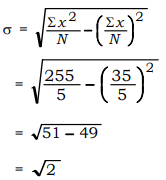σ = 1.414

23. Solve x2 – 2x – 4 = 0 by using formula.

Solution:

x2 – 2x – 4 = 0

Comparing with the standard form ax2 + bx + c = 0,

a = 1, b = -2, c = -4

x = [-b ± √(b2 – 4ac)]/2a

x = [-(-2) ± √{(-2)2 – 4(1)(-4)}]/ 2(1)

= [2 ± √(4 + 16)]/2

= (2 ± 2√5)/2

= 2(1 ± √5)/2

= 1 ± √5

Hence, the roots of the given equation are (1 + √5) and (1 – √5).

OR

Determine the nature of the roots of the equation x2 – 2x – 3 = 0.

Solution:

x2 – 2x – 3 = 0

Comparing with the standard form ax2 + bx + c = 0,

a = 1, b = -2, c = -3

Δ = b2 – 4ac

= (-2)2 – 4(1)(-3)

= 4 + 12

= 16

Δ > 0

Discriminant is greater than 0.

Therefore, the roots of the equation are real and distinct.

24. In a circle of radius 3·5 cm, draw two radii such that the angle between them is 80°. Construct tangents to the circle at the non-centre ends of the radii.

Solution:

Given,

Angle between two radii = 80°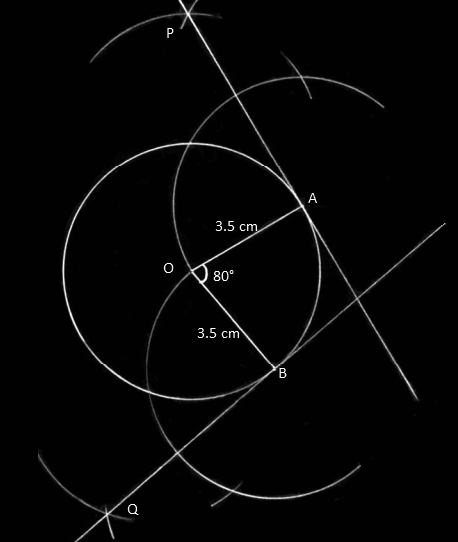25. In ΔABC, D is a point on BC such that ∠BAC = ∠ADC. Prove that AC2 = BC × DC.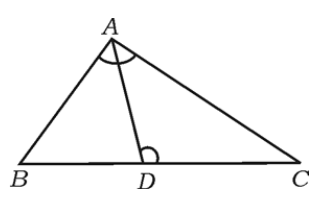Solution:

∠ACB = ∠ACD (common)

By AA similarity criterion,

ΔACB ~ ΔDCA

⇒ AC/DC = CB/CA

⇒ AC2 = BC × DC

Hence proved.

OR

In the right triangle ABC, ∠ABC = 90° and BD ⊥ AC. Prove that: AB2/BC2 = AD/CD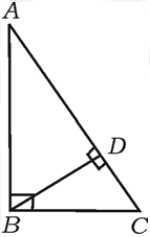Solution:

Given that, in right triangle ABC, ∠ABC = 90° and BD ⊥ AC.

By the corollary of right angle theorem,

AB2 = AD × AC ….(i)

And

BC2 = CD × AC ….(ii)

Dividing (i) by (ii),

AB2/BC2 = (AD × AC)/ (CD × AC)

Hence proved.

26. Find the value of sin 30° . cos 60° – tan245°.

Solution:

sin 30° = 1/2

cos 60° = 1/2

tan 45° = 1

sin 30° . cos 60° – tan245°

= (1/2) × (1/2) – (1)2

= (1/4) – 1

= (1 – 4)/4

= -3/4

27. Find the radius of a circle whose centre is (-5, 4) and which passes through the point (-7, 1).

Solution:

Given,

Centre = C(-5, 4)

Circle passes through the point A = (-7, 1)

Radius of the circle = Distance between A and C

Let, (x1, y1) = (-5, 4)

(x2, y2) = (-7, 1)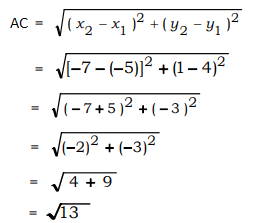Therefore, the radius of the circle is √13 units.

28. The radii of two right circular cylinders are in the ratio 2 : 3 and the ratio of their curved surface areas is 5 : 6. Find the ratio of their heights.

Solution:

Let h1 and h2 be the heights of two right circular cylinders.

Given,

Ratio of the radii of two right circular cylinders = r1 : r2 = 2 : 3

Ratio of the curved surface areas = S1 : S2 = 5 : 6

2πr1h1/2πr2h2 = 5/6

2h1/3h2 = 5/6

h1/h2 = (5 × 3)/(6 × 2)

h1/h2 = 5/4

Hence, the required ratio is 5 : 4.

29. The radius of a solid metallic sphere is 10 cm. It is melted and recast into small cones of height 10 cm and base radius 5 cm. Find the number of small cones formed.

Solution:

Given,

Radius of solid sphere = R = 10 cm

Base radius of cone = r = 5 cm

Height of cone = h = 10 cm

Number of small cones = Volume of sphere/Volume of one small cone

= (4/3)πR3/ [(1/3)πr2h]

= (4 × 10 × 10 × 10)/ (5 × 5 × 10)

= 4 × 2 × 2

= 16

Hence, the number of small cones formed from the sphere = 16

30. Draw a plan by using the information given below:

[Scale: 25 metres = 1 cm]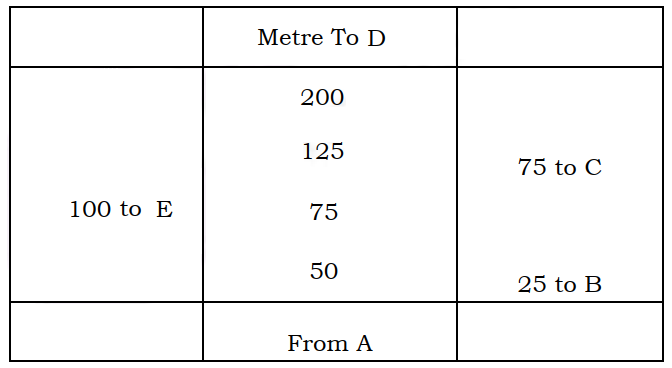Solution:

Scale:

25 m = 1 cm

50 m = 2 cm

75 m = 3 cm

100 m = 4 cm

125 m = 5 cm

200 m = 8 cm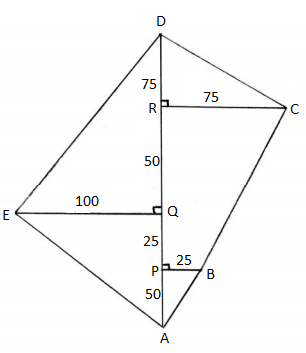SECTION – IV

31. Rationalise the denominator and simplify:

(√6 + √3)/(√6 – √3)

Solution:

(√6 + √3)/(√6 – √3)

By rationalising the denominator,

= [(√6 + √3)/(√6 – √3)] × [(√6 + √3)/(√6 + √3)]

= (√6 + √3)2/ [(√6)2 – (√3)2]

= (6 + 3 + 2√6√3)/ (6 – 3)

= (9 + 2√18)/3

= (9 + 6√2)/3

= 3(3 + 2√2)/3

= 3 + 2√2

32. Find the quotient q(x) and remainder r(x) on dividing p(x) = x3 + 4x2 – 5x + 6 by g(x) = x + 1 and hence verify p(x) = [g(x) × q(x)] + r(x).

Solution:

Given,

p(x) = x3 + 4x2 – 5x

g(x) = x + 1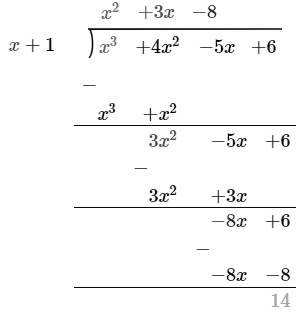Quotient = q(x) = x2 + 3x – 8

Remainder = r(x) = 14

Verification:

[g(x) × q(x)] + r(x)

= (x + 1)(x2 + 3x – 8) + 14

= x3 + 3x2 – 8x + x2 + 3x – 8 + 14

= x3 + 4x2 – 5x + 6

= p(x)

Therefore, p(x) = [g(x) × q(x)] + r(x)

OR

Find the quotient and remainder by using synthetic division:

(4x3 – 16x2 – 9x – 36) ÷ (x + 2)

Solution:

(4x3 – 16x2 – 9x – 36) ÷ (x + 2)

Using synthetic division,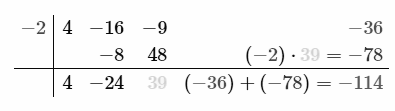Therefore,

Quotient = q(x) = 4x2 – 24x + 39

Remainder = r(x) = -114

33. Find three consecutive positive integers such that the sum of the square of the first integer and the product of the other two is 92.

Solution:

Let x, (x + 1) and (x + 2) be the three consecutive positive integers.

According to the given,

x2 + (x + 1)(x + 2) = 92

x2 + x2 + 2x + x + 2 = 92

2x2 + 3x + 2 – 92 = 0

2x2 + 3x – 90 = 0

2x2 – 12x + 15x – 90 = 0

2x(x – 6) + 15(x – 6) = 0

(x – 6)(2x + 15) = 0

x – 6 = 0, 2x + 15 = 0

x = 6, x = -15/2

x cannot be negative.

Therefore, x = 6

Hence, the required three consecutive positive integers are 6, 7 and 8.

OR

Sum of the squares of any two numbers is 180. If the square of the smaller number is equal to 8 times the bigger number, find the two numbers.

Solution:

Let x, y be the two numbers and x > y.

Sum of the squares of two numbers is 180.

i.e. x2 + y2 = 180 ….(i)

Given that the square of the smaller number is equal to 8 times the bigger number.

i.e. y2 = 8x ….(ii)

From (i) and (ii),

x2 + 8x = 180

x2 + 8x – 180 = 0

x2 + 18x – 10x – 180 = 0

x(x + 18) – 10(x + 18) = 0

(x + 18)(x – 10) = 0

x = -18, x = 10

x = -18 is not possible.

Thus, x = 10

Substituting x = 10 in (ii),

y2 = 8 × 10

= 80

y = √80 = 4√5

Hence, the required numbers are 10 and 4√5.

34. Prove that “If two circles touch each other externally, the centres and the point of contact are collinear”.

Solution:

Let A be the centre of circle C1 and B be the centre of circle C2.

P be the point of contact.

Draw tangent XY which passes through P.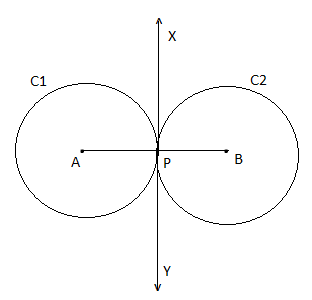∠APX = ∠BPX = 90° (radius is perpendicular to the tangent through the point of contact)

Now,

∠APX + ∠BPX = 90° + 90° = 180°

180° is the angle formed by a straight line.

Thus, ∠APX and ∠BPX is a linear pair.

Therefore, A, P and B are collinear.

Hence proved.

35. In an equilateral triangle ABC, AN ⊥ BC, prove that 4AN2 = 3AB2.

Solution:

Given that in an equilateral triangle ABC, AN ⊥ BC.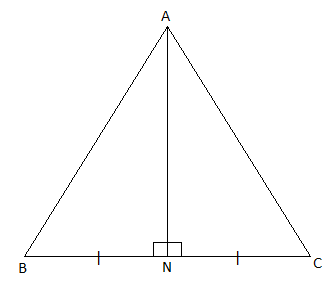Thus, BN = NC = (1/2)BC = (1/2)AB

In right triangle ANB,

AB2 = AN2 + BN2

AN2 = AB2 – BN2

= AB2 – [(1/2)AB]2

= AB2 – (AB2/4)

= (4AB2 – AB2)/4

4AN2 = 3AB2

Hence proved.

OR

In ΔABC, AD ⊥ BC, prove that AB2 + CD2 = AC2 + BD2.

Solution:

Given that in ΔABC, AD ⊥ BC.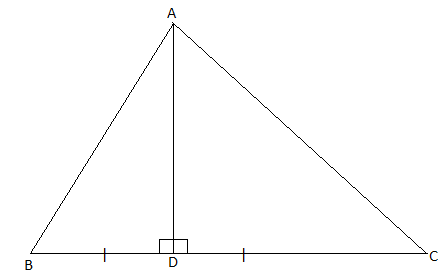⇒ AD2 = AB2 – BD2 ….(i)

⇒ AD2 = AC2 – DC2 ….(ii)

From (i) and (ii),

AB2 – BD2 = AC2 – DC2

AB2 + DC2 = AC2 + BD2

Hence proved.

36. Prove that tan2A – sin2A = tan2A . sin2A.

Solution:

LHS = tan2A – sin2A

= (sin2A/cos2A) – sin2A

= (sin2A – sin2A cos2A)/cos2A

= sin2A(1 – cos2A)/cos2A

Using the identity sin2θ + cos2θ = 1,

= (sin2A sin2A)/cos2A

= (sin2A/cos2A) sin2A

= tan2A sin2A

= RHS

Hence proved.

OR

From the top of a building 50√3 m high the angle of depression of an object on the ground is observed to be 30°. Find the distance of the object from the foot of the building.

Solution:

Let AB be the building and C be the object.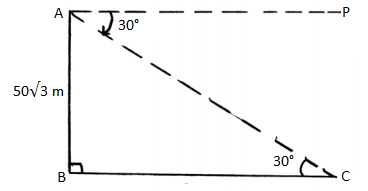AB = 50√3 m

∠PAC = ∠ACB = 30°

Angle of depression = θ = 30°

In right triangle ABC,

tan 30° = AB/BC

1/√3 = (50√3)/BC

BC = (50√3) × √3

= 50 × 3

= 150

Hence, the distance between the building and the objec = BC = 150 m

SECTION – V

37. The sum of 3rd and 5th terms of an arithmetic progression is 30 and the sum of 4th and 8th terms of it is 46. Find the arithmetic progression.

Solution:

Let a be the first term and d be the common difference of an AP.

According to the given,

a3 + a5 = 30

a + 2d + a + 4d = 30

2a + 6d = 30

2(a + 3d) = 30

a + 3d = 15 ….(i)

And

a4 + a8 = 46

a + 3d + a + 7d = 46

2a + 10d = 46

2(a + 5d) = 46

a + 5d = 23 ….(ii)

Subtracting (i) from (ii),

a + 5d – (a + 3d) = 23 – 15

2d = 8

d = 8/2 = 4

Substituting d = 4 in (i),

a + 3(4) = 15

a + 12 = 15

a = 15 – 12

a = 3

a + d = 3 + 4 = 7

a + 2d = 3 + 2(4) = 11

a + 3d = 3 + 3(4) = 15

Hence, the required AP is 3, 7, 11, 15,…

OR

If the fourth term of a geometric progression is 8 and its eighth term is 128, find the sum of the first ten terms of the progression.

Solution:

Given that in GP,

a4 = 8

ar3 = 8 ….(i)

And

a8 = 128

ar7 = 128 ….(ii)

Dividing (ii) by (i),

ar7/ar3 = 128/8

r4 = 16

r4 = (2)4

r = 2

Substituting r = 2 in (i),

a(2)3 = 8

8a = 8

a = 8/8 = 1

Sum of the first n term

Sn = a(rn – 1)/(r – 1)

S10 = 1(210 – 1)/(2 – 1)

= 1024 – 1

= 1023

Hence, the sum of the first 10 terms of the GP is 1023.

38. Solve x2 – 2x – 3 = 0 graphically.

Solution:

Given,

x2 – 2x – 3 = 0

x2 – (2x + 3) = 0

Thus, the solution will be the intersection of y = x2 and y = 2x + 3

Consider, y = x2

 x -3 -2 -1 0 1 2 3 y 9 4 1 0 1 4 9

y = 2x + 3

 x -1 0 1 2 3 y 1 3 5 7 9

Graph: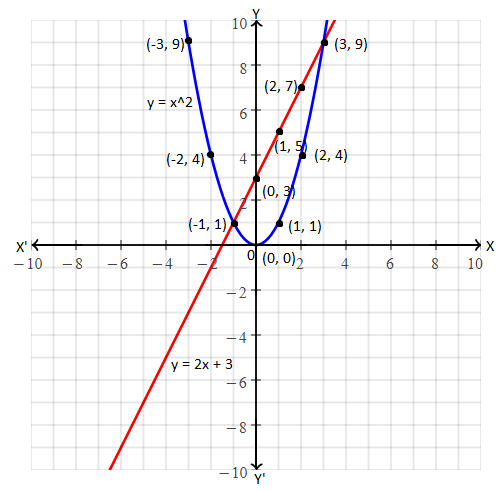These intersect each other at x = -1 and x = 3.

Hence, the required solution is x = -1 and x = 3

39. Construct a pair of direct common tangents to two circles of radii 4 cm and 2 cm whose centres are 8 cm apart. Measure and write the length of the direct common tangent.

Solution: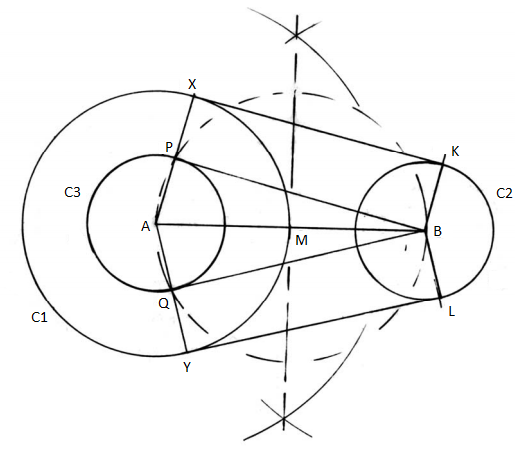Therefore, KX and LY are the required tangents of length 7.8 cm.

40. Prove that “If two triangles are equiangular, then their corresponding sides are in proportion”.

Solution:

Given,

In ΔABC and ΔDEF,

∠BAC = ∠EDF

∠ABC = ∠DEF

To prove: AB/DE = BC/EF = AC/DF

Construction:

Points G and H are marked on AB and AC such that AG = DE and AH = DF. Join GH.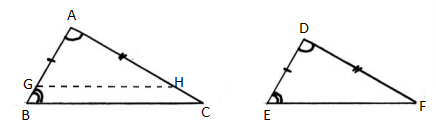In ΔAGH and ΔDEF,

AG = DE (by construction)

∠GAH = ∠EDF (given)

AH = DF (by construction)

BY SAS congruence criterion,

ΔAGH ≅ ΔDEF

Thus, by CPCT,

GH = EF

∠AGH = ∠DEF

Given that, ∠DEF = ∠ABC

∠AGH = ∠ABC (alternate angles)

Therefore, GH || BC

By the corollary of Thales(BPT) theorem,

AB.AG = BC/GH = AC/AH

And, AG = DE , GH = EF, AH = DF

Therefore,

AB/DE = BC/EF = AC/DF

Hence proved.